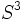# Special orthogonal group over reals

## Definition

For any natural number$n$, the special orthogonal group over reals of degree$n$, denoted$SO(n,\R)$ or$SO_n(\R)$, is defined as the following group:$\{ A \in GL(n,\R) \mid \det(A) = 1, AA^T = I \}$

This can also be described as the group of linear transformations of$\R^n$ that are orientation-preserving and also preserve the dot product.

## Particular cases

Value of$n$ Name of group Special comments
1 trivial group none
2 circle group the only nontrivial abelian case
3 special orthogonal group:SO(3,R) the smallest non-abelian case. Also, has$S^3$ (group of unit quaternions) as a double cover
4 special orthogonal group:SO(4,R)